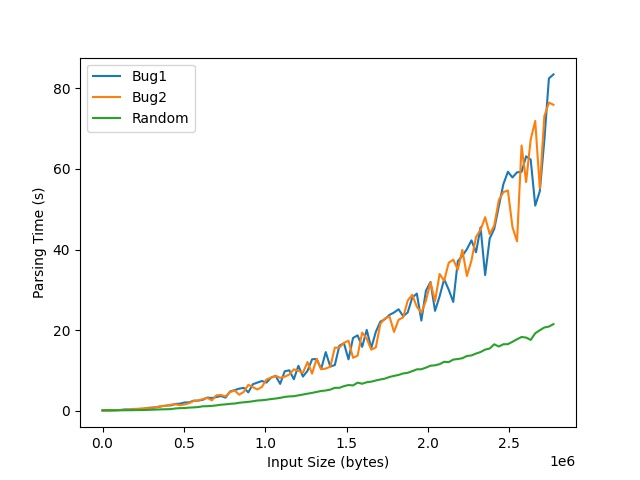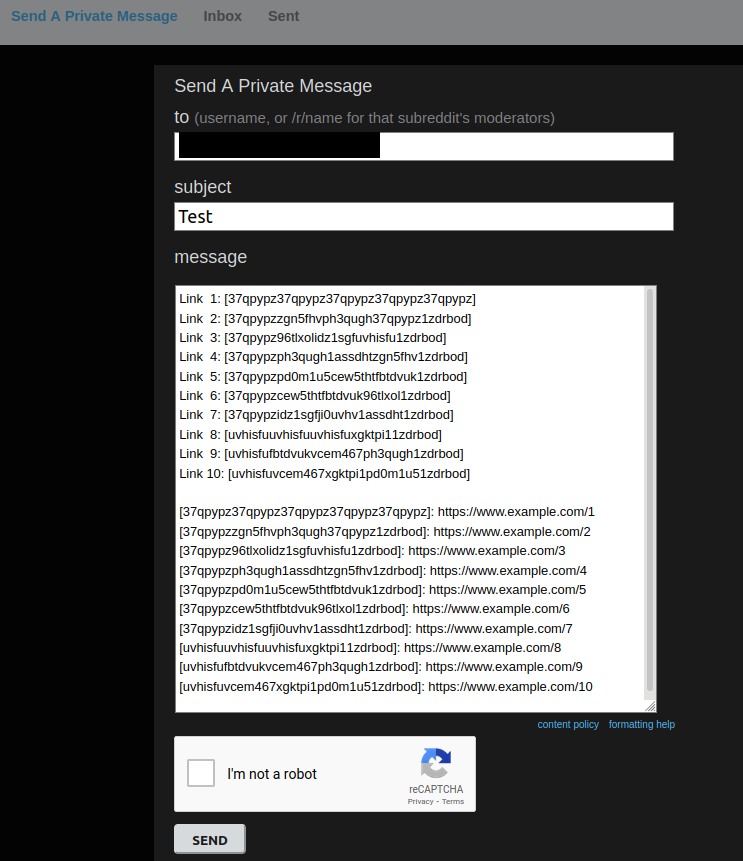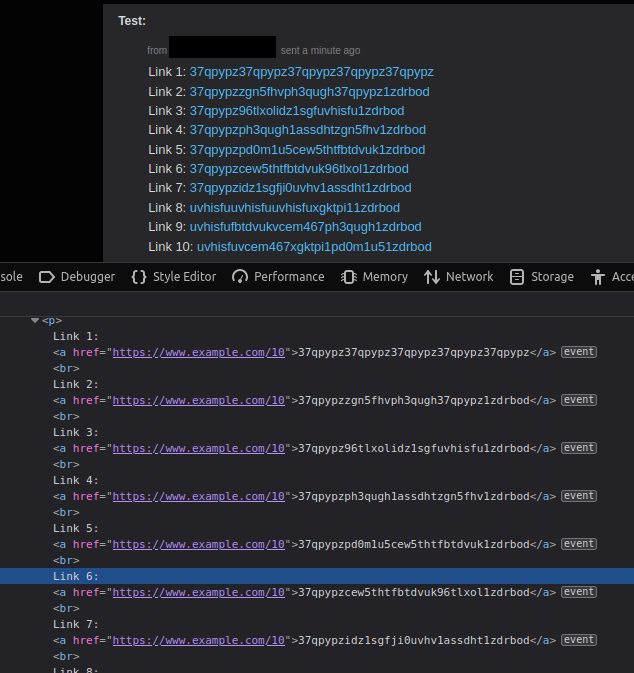## Summary:

We have found three bugs in Reddit’s markdown parser. Two of these bugs are exploitable to launch an algorithmic complexity denial-of-service (DoS) attack. In this report we explain the bugs and exploits. We also show, in a non-disruptive way, that it appears to exist in the current version of Reddit.

## Context:

Reddit uses a markdown parser to convert markdown text into HTML text. Among the features that markdown text provides, is the ability to define and use named references. In markdown, a reference is defined with the following syntax: [reference_name]: https://www.example.com A reference can be used with the following syntax: [reference_name] The resulting HTML text, will contain a link to https://www.example.com with the text reference_name: <a href="https://www.example.com/">reference_name</a>

In the backend, this feature is implemented with a hash table data structure that maps the reference name (the key) to the associated URL (the value). When a reference is defined, a hash function is used to convert the reference name into an integer (the hash value), which is mapped to an index in an array, by taking the modulus with the array length. The reference is inserted in the array at this index (code). As there may be references with different names but identical hash values (known as collisions) a linked list is kept at each index in the array. Each collision is inserted at the start of the linked list at the index it maps to.

When a reference is used, the hash value and array index are calculated again. Then, the linked list is iterated until a reference with an identical hash value is found (code). (Note: This behavior is faulty, see Bug 3).

## Bugs:

### Bug 1: The hash table uses a weak hash function

The hash function for the reference hash table is implemented in a function named hash_link_ref. This is a case-insensitive version of the SDBM hash function. This is a weak hash function, meaning that an attacker can reliably generate a large number of collisions for it. This makes the hash table vulnerable to a hash-collision DoS attack, a type of algorithmic complexity attack. In this attack, an attacker inserts a large number of collisions into the hash table. All these references will be put into the same linked list, increasing its length. Then, when a reference is used, a very large linked list needs to be iterated to find the correct entry. As a result, the time to retrieve a reference increases linearly with the number of colliding entries (O(N), where N is the number of colliding entries). This is considered an algorithmic complexity vulnerability, as the expected behavior of a hash table is to have constant time retrieval and insertion (O(1), i.e. the retrieval and insertion time are unaffected by the number of entries in the table). These vulnerabilities can be exploited in DoS attacks.

We show a proof-of-concept exploit for this vulnerability in the Proof-of-Concepts section.

### Bug 2: The hash table allows duplicate entries

Typically, a hash table only allows unique keys. While allowing duplicate keys improves the insertion time (to O(1)) (code), it leaves the hash table vulnerable to a different algorithmic complexity attack. This attack is similar to the attack on Bug 1, but is simpler to execute, because it does not require many colliding entries. Instead, an attacker can create a long linked list with duplicate entries and trigger long retrieval time by using the reference associated with the last entry in the linked list. (This will be the first defined reference, as each definition is inserted at the start of the linked list)

We show a proof-of-concept exploit for this vulnerability in the Proof-of-Concepts section.

### Bug 3: The hash table determines key equality on hash value alone

In the hash table implementation, when a reference name is used, an entry in the hash table is retrieved by searching for a reference with the same hash value (code). This behavior is incorrect. Since there are more possible distinct reference names than hash values, there are necessarily different reference names with the same hash value (by the pigeonhole principle). This means, an incorrect reference may be retrieved for a reference name. For example, with respect to the SDBM hash function, the input strings 37qpypz and uvhisfu collide, as they both produce the hash value 7150400. Therefore, defining and using two references with these names:

Link 1: [37qpypz]
[37qpypz]: http://www.url1.com
[uvhisfu]: http://www.url2.com


Will result in the second URL being retrieved for both references, which leads to the HTML text:

Link 1: <a href="https://www.url2.com/">37qpypz</a>


This bug cannot be exploited to perform malicious actions (as far as we know), but it is useful to show that the current version of Reddit is still vulnerable to attacks on Bugs 1 and 2.

## Proof-of-Concepts:

To illustrate the vulnerabilities caused by Bugs 1 and 2, respectively, we will measure the time it takes for the markdown parser (running locally) to parse both random and malicious reference names in markdown text. The markdown text will be formatted as follows:

[s1]: /url
[s1]
[s2]: /url
[s1]
[s3]: /url
[s1]
...
[sN]: /url
[s1]


Each string si is a reference name generated either randomly or in a specific way to exploit either Bug 1 or 2. We will measure the parsing time while increasing N to show that it grows faster for the malicious input than for the random input. The reason for this is:

Each pair

[si]: /url
[s1]


contains both a reference definition and a use. Each definition will insert a new entry into the hash table and the use will retrieve the first defined reference from the table. For malicious input, every definition will insert a reference at the start of the same linked list as the first defined reference. This means, every time we use the first defined reference after a new definition, a longer linked list will need to be iterated to retrieve it.

Conversely, for random reference names, each definition will insert the reference into a random linked list in the hash table. If the randomly named reference is not inserted into the same linked list as the first defined reference, the time to retrieve the latter will be unaffected. Therefore, we expect to observe a much faster growth in parsing time for malicious input than for random input. Note, we will still observe an increase in retrieval time for randomly named references, due to randomly occurring collisions. We perform these experiments and plot the input size (x-axis) against the parsing time (y-axis). We show the graph below, which illustrates the expected behavior.Next, we explain how we generate malicious input for both attacks on Bug 1 and Bug 2. That is, how we generate s1, s2, … , sN.

### Bug 1:

In the typical hash-collision DoS attack, one would simply generate a large number of collisions for the hash function. However, due to Bug 3, this will not work in this case, since the first item in the linked list will be returned (in time O(1)) as it has the same hash value. Therefore, instead, we find many non-colliding strings, s1, s2, …, sN such that: hash(si) != hash(sj) when i != j, but (hash(si) % T) == (hash(sj) % T) for all i and j, where T is the hash table size. In other words, each string has a unique hash value, but they are all still inserted into the same linked list, because they map to the same table index after taking the modulus with the table size.

### Bug 2:

Generating malicious input to exploit Bug 2 is significantly easier. We only find two strings s’ and s’’ such that hash(s’) != hash(s’’) but (hash(s’) % T) == (hash(s’’) % T) Then, let s1 = s’ and s2 = s’’, s3 = s’’, …, sN = s’’. Since the hash table does not prevent us from entering string s’’ multiple times, we can increase the linked list length by reusing the same string.

## Steps to Reproduce:

We need a non-disruptive way to show that the bugs exist in Reddit. To this end, we use Bug 3. Since the hash table considers reference names with the same hash value to be equal, the first entry in the linked list with the correct hash value will be returned. We can confirm that SDBM hash is used by the current version, by using a small number of colliding reference names, each with a unique URL, and observing the generated HTML text. If SDBM hash is indeed used, the use of any of these references will incorrectly yield the final URL (as this is first in the linked list).

We show the setup and outcome of this experiment. In the first image, we show the markdown text we use in a private message. Note that each of the reference names point to a different URL. Each of the reference names we use collide with respect to the SDBM hash function.In the second image, we show the HTML text of the received private message, created from the markdown text. It is clear that the same URL (https://www.example.com/10) was retrieved, regardless of which reference name was requested. This is the incorrect behavior we expect if SDBM hash is used, which means Bug 1 exists in the current version of Reddit.### Fixes

The the bugs were fixed in this pull request, in the following manner.

#### Bug 1: The hash table uses a weak hash function

Bug 1 can be fixed by replacing the SDBM hash function with a more secure, keyed hash function. A number of hash functions were created to mitigate hash-collision DoS vulnerabilities, such as HighwayHash and SipHash.

##### Requirements:
• The current hash function converts reference labels to lower case while computing a hash value, to achieve case insensitivity. The replacement hash function should also compute case insensitive hash values.

#### Bug 2: The hash table allows duplicate entries

Bug 2 can be patched by updating the function that inserts references into the hash table. After retrieving the correct linked list to insert the new reference into, this linked list should first be iterated to ensure the same reference does not already exist therein.

##### Requirements:
• What the hash table considers to be the same reference should also be updated (see Fixes for Bug 3).

#### Bug 3: The hash table determines key equality on hash value alone

When iterating the linked list to determine if a reference exists in it, the reference labels should be compared, not only the hash values.

##### Requirements:
• Two reference labels should be compared in a case insensitive manner, to match the behavior of the hash function.